#### You may also like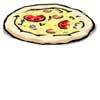### Pizza Portions

My friends and I love pizza. Can you help us share these pizzas equally?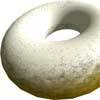### Doughnut

How can you cut a doughnut into 8 equal pieces with only three cuts of a knife?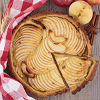### Pies

Grandma found her pie balanced on the scale with two weights and a quarter of a pie. So how heavy was each pie?

# In the Money

##### Age 7 to 11Challenge Level

We had a very good number of correct solutions sent in with a variety of explanations.

Oliver from Peak School in Hong Kong said:

First, I thought of the numbers that are multiples of both 3 and 4, so I tried doing 12.
However, if three coins were heads, which is essential because that is one-quarter of 12, then if I turned two more heads, then it would make five coins heads which are not a third of 12.
Next, I tried doing 24. A quarter of 24 is 6 and 6 and 2 is 8. 8 is a third of 24, so that is how I got the answer.
The methods that I used during this activity is logical thinking and guess, check and improve.

Hayden, also from Peak wrote:

First I thought about multiples of 3 and 4 and which numbers are in both multiples.
So I tried 12 at first but a quarter of 12 is 3 and then if you add 2 it's 5 and that is not a third of 12.
Next, I tried 24 so a quarter is 6 and if you add 2 it's 8. And that is a third of 24. The method I used is guess, check and improve. I think this is a useful method because in this problem it wouldn't be efficient to just test all the number and logically that would take a long time to reach 24.

Also from Peak, Iris and Elena said:

The first thing we tried was to write a list of the multiples of 3 and 4 and tried to match them up, we tried 12 because both 4 and 3 go into 12 but that didn't work.
Then we realized it had to be bigger than 12 because you can't let the quarter get too big (compared to the number) and become more than one third, so then we experimented with a couple of numbers and finally landed on 24.
So there were 24 coins on the table and 6 had the heads shown we flipped two of the tails over and that made 8 which is one third of 24.

Rishi from Orton Wistow Primary School wrote:

It is 24 because the difference between $\frac{1}{4}$ and $\frac{2}{3}$ is $\frac{1}{12}$ which means that two coins are $\frac{1}{12}$ so 2 x 12 is 24. I didn't think like the children.

Aadi from Coldspring sent in the following:

I figured out that there are twenty-four coins. One quarter of 24 is 6 and one third of 24 is 8. Eight times three equals twenty-four. Six times four is twenty-four. You start out with six coins facing heads up. Then you flip over two more coins and you get eight coins that are facing heads up. The amount of coins is twenty-four.

Chen from ISL in China wrote:

I've known that $\frac{1}{4}$ = $\frac{3}{12}$, $\frac{1}{3}$ = $\frac{4}{12}$.
$\frac{1}{12}$=2 coins, so the number of the coins was: 2x12=24coins

Lauren, Eliya and Hannah from North London Collegiate Junior School said:

If you start with a quarter of the coins as heads and turn over two to have as heads, then one third of the coins takeaway one quarter of the coins = 2
$\frac{1}{3}$ - $\frac{1}{4}$ = $\frac{4}{12}$ - $\frac{3}{12}$ = $\frac{1}{12}$ of the coins = 2 coins
If 2 coins are $\frac{1}{12}$ of the coins then the total number of coins is 24.
If there were 24 coins and a one quarter were heads then there were 6 heads. If you turn over two tails then you have 8 heads, which is one third of 24.

From Kingsfleet School in Felixstowe, Malakai, George, Joshua and Freya sent in the following.
Their different approaches can be viewed larger on a new page by clicking on the images.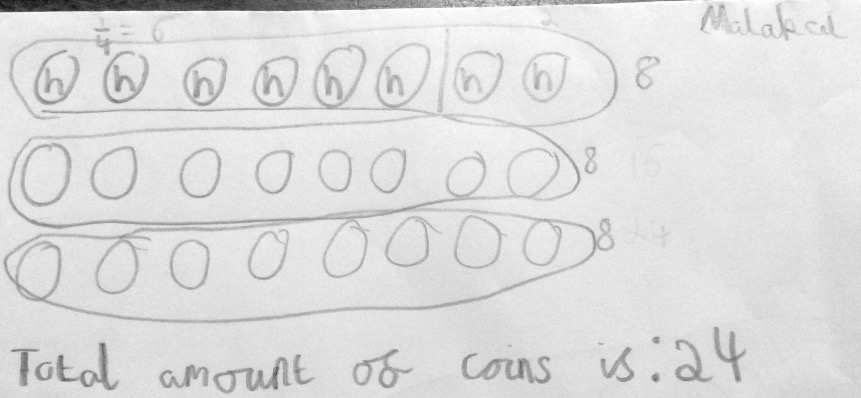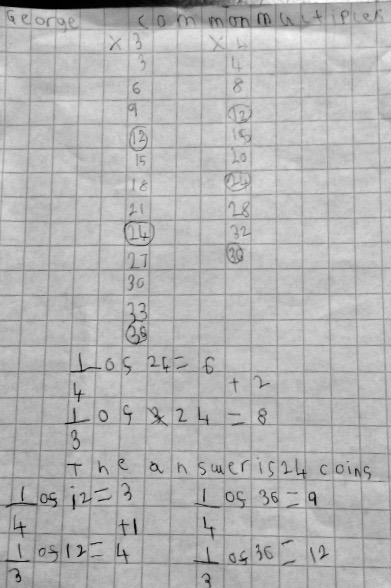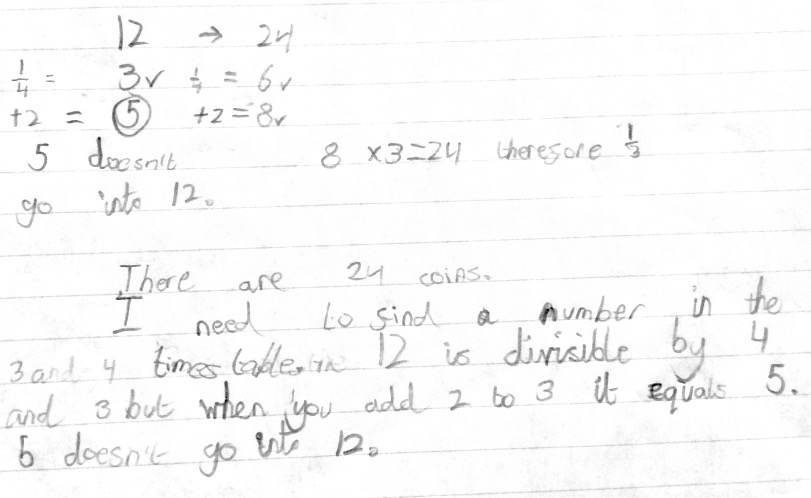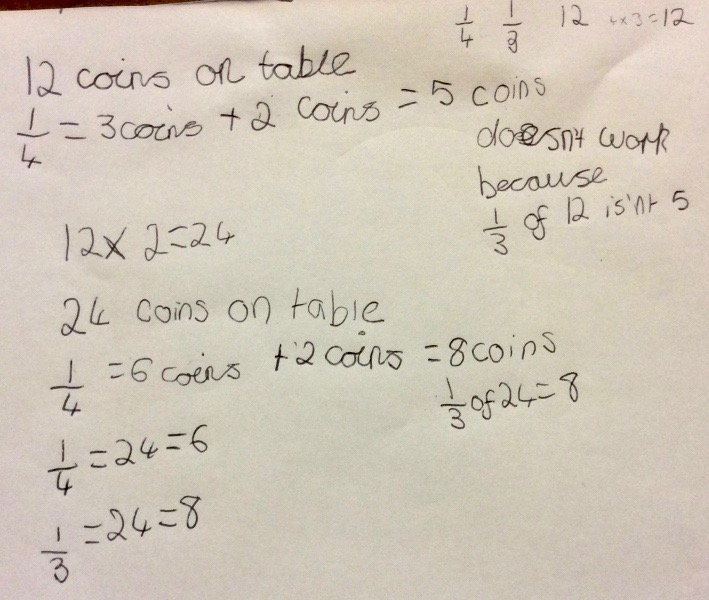Students from Brussels International School also sent in their different approaches, which can be viewed larger on a new page by clicking on the images:

Jack R, Elise, Evie, Leo and Audrey: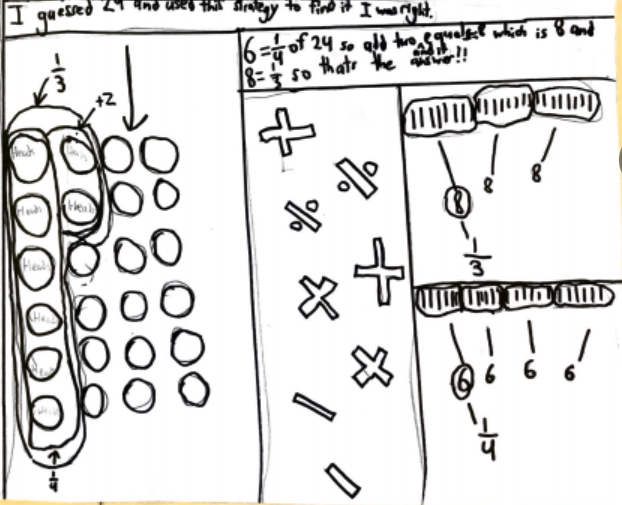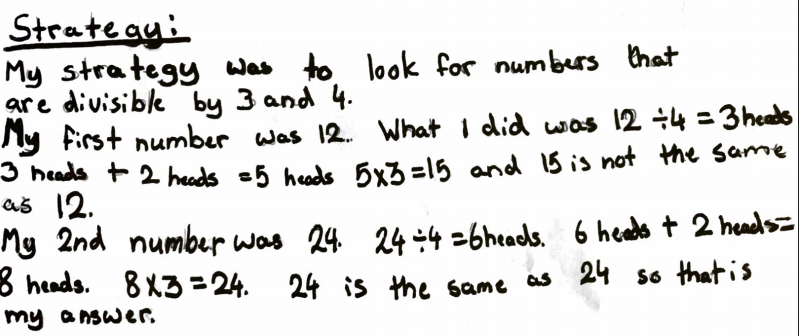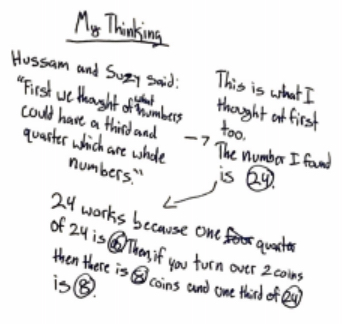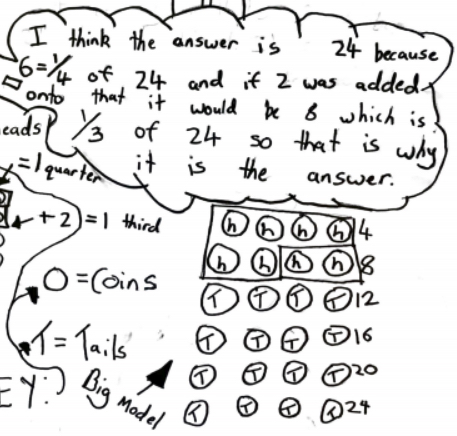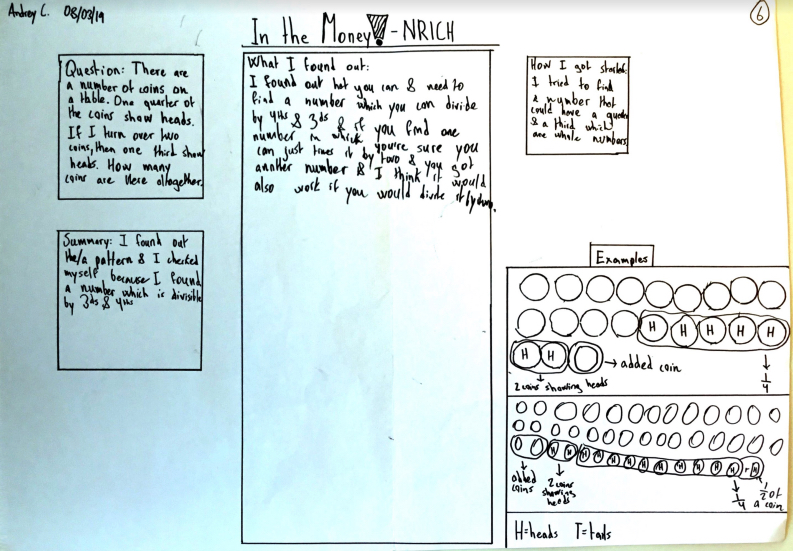Ben, Cécilia, Eleanor, Jack and Julia: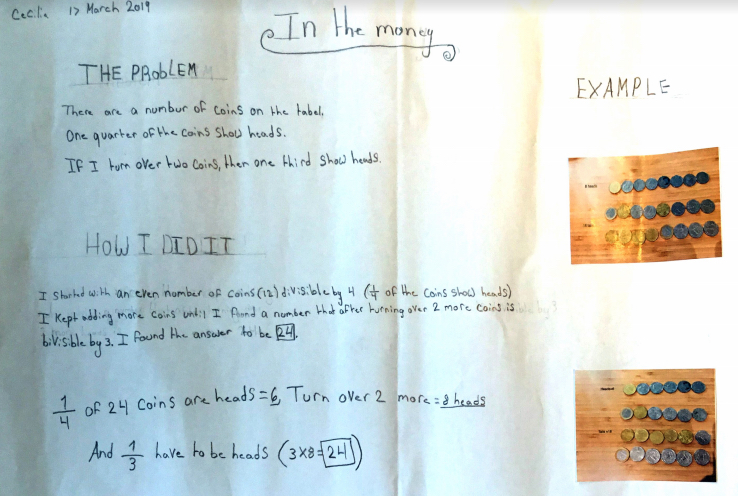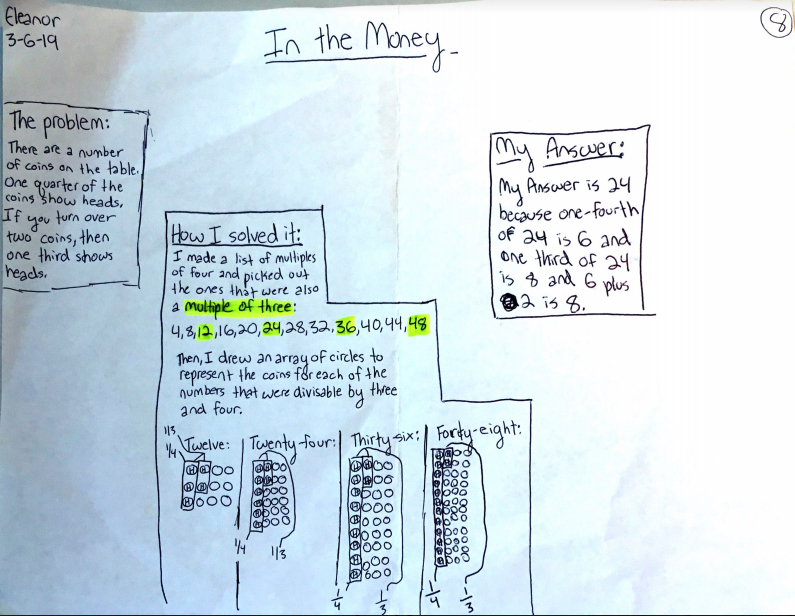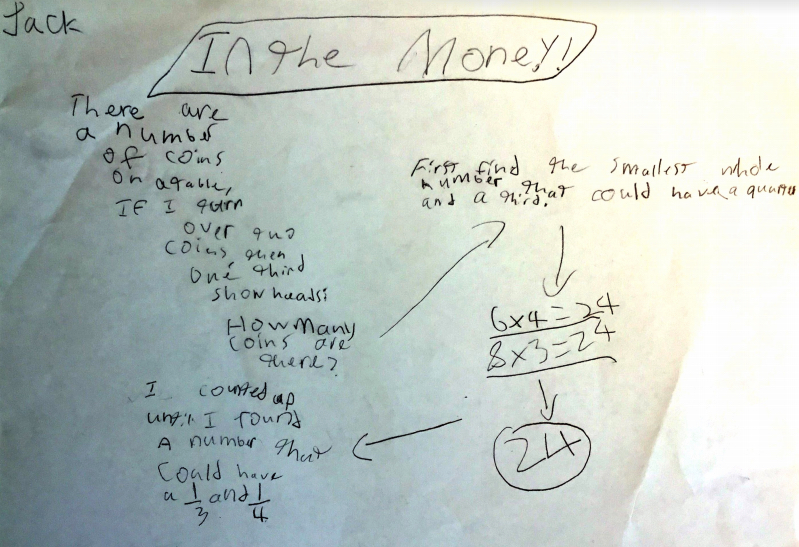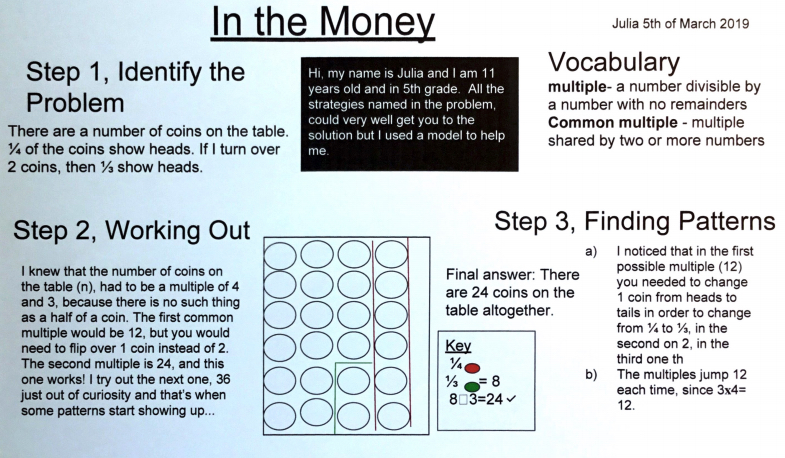Kyuwon, Marina, Quinn and Téa: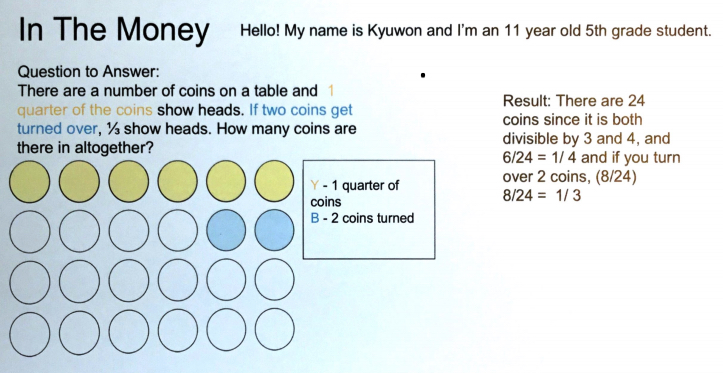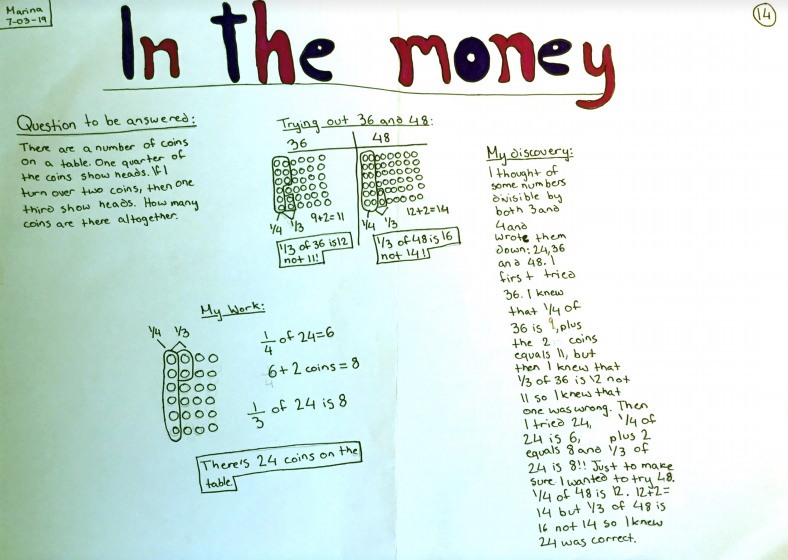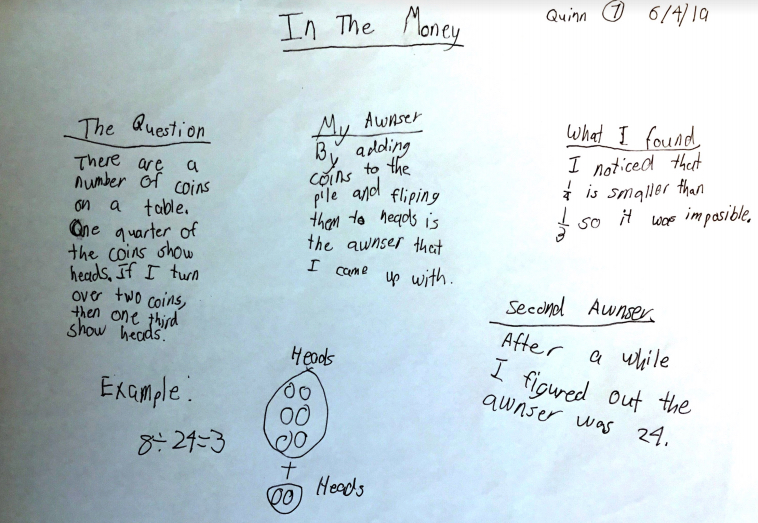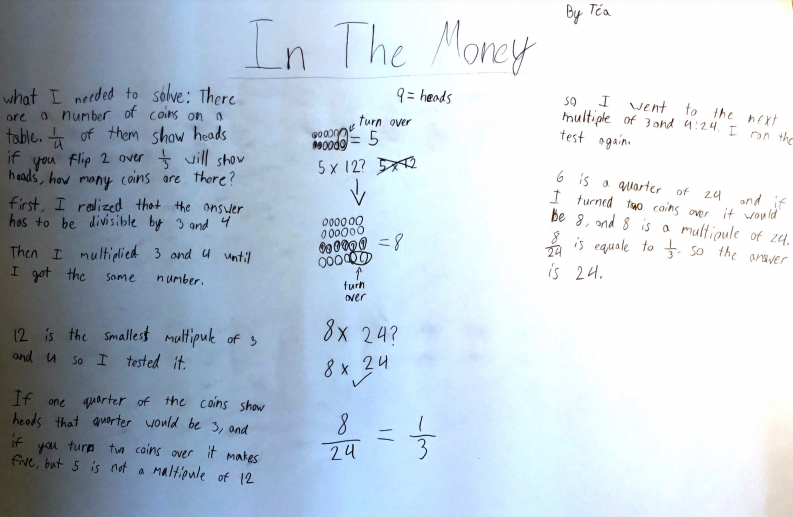Florence from Walthamstow Hall Junior School wrote:

I started off by seeing what numbers under 30 were in both the 3 and 4 times tables. Under 30 because with any number over that, turning over just 2 was unlikely to make a difference. (You could always carry on afterward if it didn't work.) The answers are 12 and 24.
If the answer was 12: There would be 3 heads and 9 tails. If you turn over 2 heads to make 5 heads and 7 tails... ... 1 third of 12 is 4, not 5.
If the answer was 24 There would be 6 heads and 18 tails. If you turn over 2 heads to make 8 heads and 16 tails... ... 1 third of 24 is 8!
Therefore 24 is the total amount of coins that were on the table to start off with.

Also from the same school, Emily sent in the following:

T= total number of coins
H=T/4
H+2=T/3
So by substituting H with T/4, we get T/4+2=T/3
Now we solve to find T by multiplying both sides by three
3/4T+6=T
6=1/4T
T=24
I check by this method
24/4=6
6+2=8
8x3=24

Thank you all for your good responses to this challenge. Well done everyone.